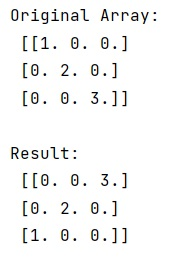# numpy.flipud() Method with Example

Learn about the numpy.flipud() method with its usage, syntax, and example.
Submitted by Pranit Sharma, on March 21, 2023

## numpy.flipud() Method

The numpy.flipud() method is used to reverse/flip the array in the up-down direction. For a 2-D array, this flips the entries in each column in the up/down direction. Rows are preserved but appear in a different order than before.

It is somewhat similar to flip but different to fliplr as flip reverses the order of an array along the given axis whereas fliplr reverses the order of an array along axis 1 that too in the left/right direction.

## Syntax

```numpy.flipud(m)
```

## Parameter(s)

• m: It takes a single parameter m which is the input array and it returns an array of flipped order.

## Return Value

It returns a view of m with the rows reversed.

## Example of numpy.flipud() method in Python

```# Import numpy
import numpy as np

# Creating a numpy array
arr =  np.diag([1.0, 2, 3])

# Display original array
print("Original Array:\n",arr,"\n")

# Flipping the array in up-down direction
res = np.flipud(arr)

# Display result
print("Result:\n",res,"\n")
```

### OutputReference: numpy.flipud()